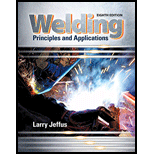Chapter 20, Problem 21R### Welding: Principles and Applicatio...

8th Edition
Larry Jeffus
ISBN: 9781305494695

#### Solutions

Chapter
Section### Welding: Principles and Applicatio...

8th Edition
Larry Jeffus
ISBN: 9781305494695
Textbook Problem
1 views

# When the denominators of two fractions to be added or subtracted are different, what must be done before they can be added?

To determine

Addition or subtracted of fraction numbers.

Explanation

Two fractions are directly added if the denominators of both the numbers are same.

If denominators of both the numbers are not same, then first convert the denominators of both the fractions.

To convert a denominator, multiply both numerator and denominator of the fraction by the same number.

When denominators converted into same numbers then added or subtracted directly numerator to numerator and denominator to denominator. For example

Example: - Addition of two numbers 1/2 and 1/6,

S=12+16S=12×33+16S=36+16S=3+16

### Still sussing out bartleby?

Check out a sample textbook solution.

See a sample solution

#### The Solution to Your Study Problems

Bartleby provides explanations to thousands of textbook problems written by our experts, many with advanced degrees!

Get Started

#### Determine the angle at which the bar AB is in equilibrium. Neglect friction.

International Edition---engineering Mechanics: Statics, 4th Edition

#### Briefly define quality control and explain its purpose.

Precision Machining Technology (MindTap Course List)

#### Name the two basic types of compression gauges.

Automotive Technology: A Systems Approach (MindTap Course List)